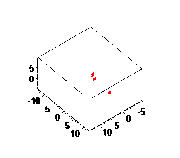## FANDOM

580 PagesThe conclusions here about gravity can allow one to make sense of the animation above.

## An intervening mass may act to either bring two masses closer together or to take them farther apart. But how?Edit

### Basic problemEdit

Consider three masses (varied in mass) separated by a distance.

The first mass is located at (-1,0). The second mass is located at (1,0).

For what (x,y) would a third mass compress the second and first together?

As long as the third mass is between x=-1 and x=1, the third mass will cause a collapse between the two objects.

### Intermediate problemEdit

Let's change the problem a bit.

When would the second mass cause a compression between the first mass and the third mass?

Define the slope between the first and third masses.

The equation y=mx+b:

Use x and y for both the first and third masses to solve for both b's. For each solution, calculate the y's for x=1. When the y-value of the second mass is between those two y values, the second mass will cause convergence of the first and third masses. It must be between in order to fit within the desired range.

By running the simulation, one can show that in most configurations, the presence of the second mass helps to prevent the first and third masses from colliding.

## The kinetic energy of one planet be transferred to another through gravitational means. But how?Edit

### Gravitational collapseEdit

Observations of stellar rebirth certainly prove this to be the case. The question is, what can be said of the elasticity of the interactions between gravitating bodies?

When it comes to electromagnetism, increasing proximity of like charges increases potential energy, which by definition absorbs kinetic energy. The like charges will repel under pressure. On the contrary, increasing the proximity of opposite charges reduces potential energy and increases kinetic energy. It is unlikely however that the momentum of oppositely charged particles will cancel exactly, and this as well as other reasons is why atoms can exist.

When it comes to gravity however, masses only behave as though they can only attract, except at very short distances where gravity becomes insignificant, such as found in the subatomic level.

As soon as a planet moves, its gravitational field moves with it. With it, any increases in proximity to nearby planets and other masses will do work on those masses. The planets will not collide head on unless their paths cross. In doing so, they obtain a relative orbital angular momentum approaching zero, where their direction of travel is largely along the distance straight between them.

But their are other ways for kinetic energy to be converted to potential energy, and back again.

Consider the case of a large heavy body and small lighter body. When the large heavy body moves with respect to the smaller lighter body, the same magnitude of force exists on the larger heavy body that it does on the smaller lighter body. The lighter body will have a greater ability to accelerate with respect to everything else in the universe.

Because the forces are equal and opposite and because the relative velocities are opposite, there is no net power transfer from one object to the other.

But consider the following.

Let's say you have two masses with zero initial velocity relative to each other as well as an outside observer. These are two nebula, and they are about to turn around and collapse, and as soon as they do accelerate, they will then have a relative velocity with respect to each other. The first mass is twice as heavy as the second mass. With respect to the outside observer, both masses gain momentum at the same rate, due to the same forces. But because the lighter mass travels faster in relative terms, more power seems to be done on the lighter mass than the heavier mass to generate kinetic energy. So where did this kinetic energy come from?

Seemingly, there was a net transfer of kinetic energy from the larger body to the smaller body. If so, the larger body must have lost more potential energy than the smaller body.

Let's get rid of one the masses.

If we get rid of the small mass, and we still see it accelerating due to gravity, then we would say that some other mass is acting upon it. If we double the mass in place, then the forces should double, and the acceleration would remain unaffected.

Therefore, the only way to explain this is a net transfer of kinetic energy from the larger mass to smaller mass and a net transfer of potential energy from the smaller mass to the larger mass. When there are two objects accelerating towards each other through gravity, the smaller body will gain more kinetic energy, and the larger body will lose more kinetic energy. If the smaller body were to gain kinetic energy close to E=MC^2 from the large, the large body would have to lose that same amount of energy to it, but gain the same amount in terms of potential energy.

Therefore, as more gravitational contraction occurs, smaller masses will obtain more and more of the kinetic energy while larger masses will obtain more and more of the potential energy.

Community content is available under CC-BY-SA unless otherwise noted.